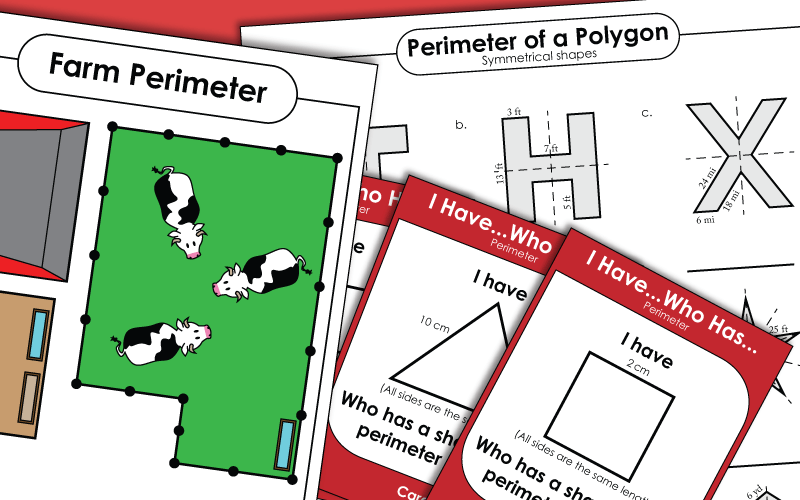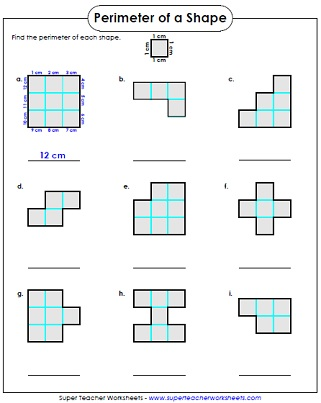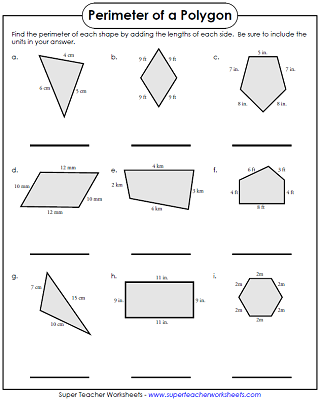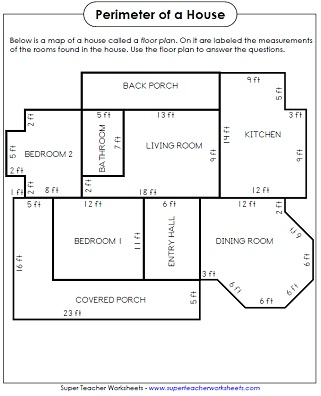# Perimeter Worksheets

Add the lengths of the sides to determine the perimeter of the polygons on these worksheets.## Basic(Simple Shapes & Whole Numbers)

Add the lengths of the sides of each shape to find the perimeters.
Find the perimeter of each shape by counting the centimeters along the edges.
This file has 10 challenging perimeter word problems. There's one problem per page and lots of space for students to show their work.
Play this whole-class card game to review perimeter. Each student must calculate the perimeter on his card and read it aloud on his turn.
Scoot! is a game in which students move around to each desk in the classroom while finding the perimeter of polygons.
Measure the lengths of the sides of the corn field, the pigs' pen, and the cows' field.  Add the sides to find the perimeter.
Basic introduction to perimeter.  Students add the sides of the polygons.  Recommended for grades 3 and up.

## Intermediate

Determine the lengths of the missing sides, then calculate the perimeter.  Grades 4 and up.
Read the floor plan to find the perimeter of each room in the house.
Find the perimeter by adding the length of the sides of each polygon.  This worksheet has larger numbers than the worksheet above and not all sides are labeled.  Recommended for grades 4 and up.
Count the lengths of the sides to determine the perimeter of each shape. 4th grade and higher.

Add the sides of the polygons to find the perimeters.  Includes decimals and fractions.
Find the perimeter of each shape. Some polygons have sides with unknown lengths that students must calculate. Includes whole numbers, fractions, and decimals.
Students use their knowledge of symmetry to find the perimeter of each figure shown.

## Perimeter & Area Mixed

Add and multiply to find the perimeters and areas of these rectangles.
Count the square centimeters to find the area and the perimeter of the shapes.
Area Worksheets

Worksheets in which students calculate the area of the given shapes.

Geometry Worksheets

All types of geometry skills, including polygons, lines, angles, symmetry, congruent shapes, and more.

Circumference Worksheets

Circumference and area of a circle, as well as a basic introduction to radius and diameter.

## Sample Worksheet ImagesMy Account
Site Information